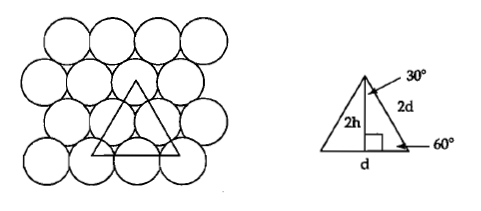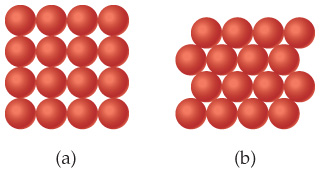# Problem: The diameter of a rubidium atom is 4.95 Å. We will consider two different ways of placing the atoms on a surface. In arrangement (a), all the atoms are lined up with one another. Arrangement (b) is called a close-packed  arrangement because the atoms sit in the "depressions" formed by the previous row of atoms: How many Rb atoms could be placed on a square surface that is 5.5 cm on a side, using arrangement (b)?

###### FREE Expert Solution

Let's consider the triangle shown below:• This is an isosceles triangle with a side-length of 2d and an angle of 60°.
• Drop a bisector to the uppermost angle so that it bisects the opposite side, the result is a right triangle with two known side lengths.
93% (227 ratings)###### Problem Details

The diameter of a rubidium atom is 4.95 Å. We will consider two different ways of placing the atoms on a surface. In arrangement (a), all the atoms are lined up with one another. Arrangement (b) is called a close-packed  arrangement because the atoms sit in the "depressions" formed by the previous row of atoms:How many Rb atoms could be placed on a square surface that is 5.5 cm on a side, using arrangement (b)?

Frequently Asked Questions

What scientific concept do you need to know in order to solve this problem?

Our tutors have indicated that to solve this problem you will need to apply the Dimensional Analysis concept. You can view video lessons to learn Dimensional Analysis. Or if you need more Dimensional Analysis practice, you can also practice Dimensional Analysis practice problems.